# Clock hands

The second hand has a length of 1.5 cm. How long does the endpoint of this hand travel in one day?

Correct result:

s =  13571.6803 cm

#### Solution: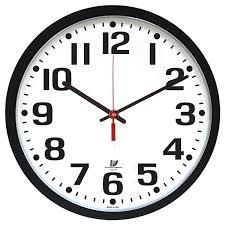We would be pleased if you find an error in the word problem, spelling mistakes, or inaccuracies and send it to us. Thank you!#### You need to know the following knowledge to solve this word math problem:

We encourage you to watch this tutorial video on this math problem:

## Next similar math problems:

• Minute-hand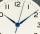How long distance will travel a large (minute) hand on the clock for 48 minutes, if its length is 56 cm?
• ClocksHow long track travel the second hand in 46 hours, the end of which is 4 cm long.
• Clocks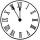What distance will describe the tip of minute hand 6 cm long for 20 minutes when we know the starting position with finally enclose hands each other 120°?
• ClocksHow long path will walk the end of the clock's minute-hand long 7 cm for 330 minutes? How long will walk in the same time hour-hand?
• HandsThe clock shows 12 hours. After how many minutes will angle between the hour and minute hand 90°? Consider the continuous movement of both hands hours.
• Clock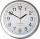How long is trajectory of second hand of hours for day, if is 15 mm long?
• Circle - easy 2The circle has a radius 6 cm. Calculate:Calculate the radius of the circle whose length is 107 cm larger than its diameter
• Coal mine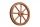The towing wheel has a diameter of 1.7 meters. How many meters does the elevator cage lower when the wheel turns 32 times?
• Bicycle wheel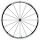Bicycle wheel diameter is 62 cm. How many times turns the bicycle on the road 1 km long?
• ParallelogramCalculate the missing side of a parallelogram, if you know the perimeter o and one side: a) o = 7.2 cm; b = 1.8 cm b) o = 5.4 cm; a = 1.9 cm
• Clock hands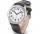The hands on the clock shows the time 12 hours and 2 minutes. Calculate the size of a sharp angle between clock hands three hours later.
• Isosceles triangleThe circumference of the isosceles triangle is 32.5 dm. Base length is 153 cm. How long is the leg of this triangle?
• Second sideCalculate the length of the other side of the rectangle if its circumference is 60 cm and one side is 10 cm long.
• Ratio - rectangleThe rectangle has dimensions 6 cm and 9 cm. How many times increases area and its perimeter, when its dimensions increase in the ratio 5:3?
• The parallelogram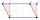The parallelogram has one side 2 dm long, which is one-sixth of its circumference. How many cm does the other side of the parallelogram measure?
• Athlete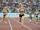How long length run athlete when the track is circular shape of radius 120 meters and an athlete runs five times in the circuit?